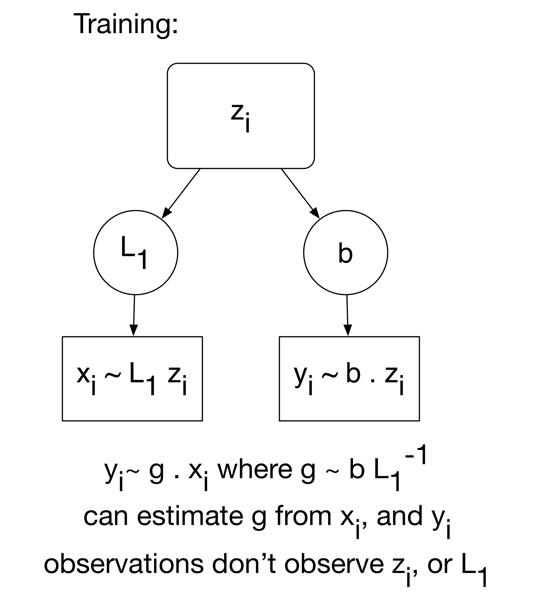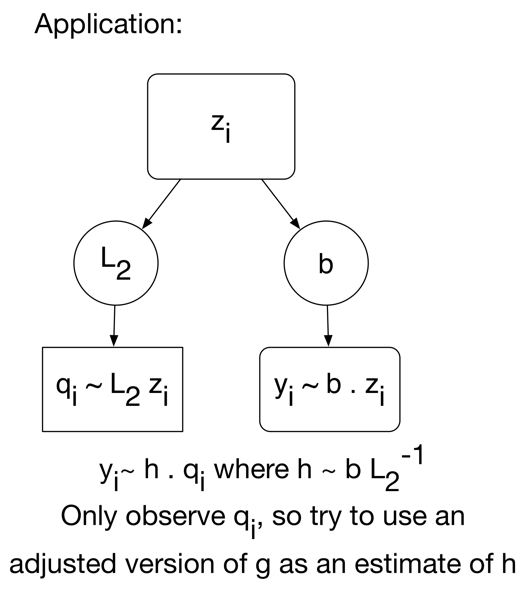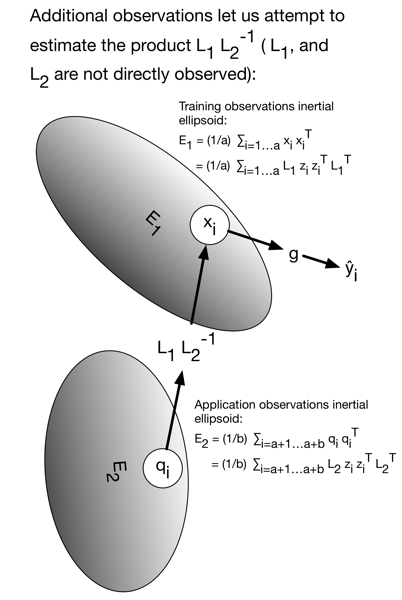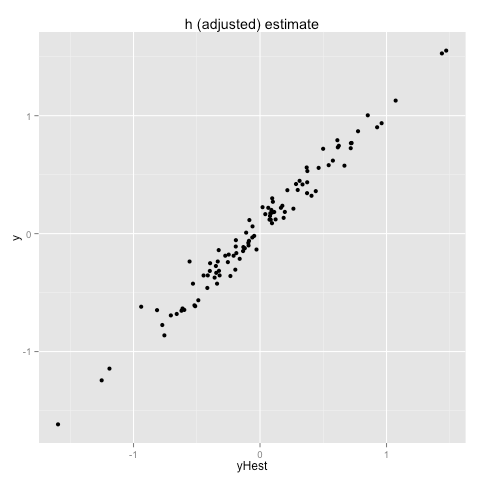# Can we try to make an adjustment?

In most of our data science teaching (including our book Practical Data Science with R) we emphasize the deliberately easy problem of “exchangeable prediction.” We define exchangeable prediction as: given a series of observations with two distinguished classes of variables/observations denoted “x”s (denoting control variables, independent variables, experimental variables, or predictor variables) and “y” (denoting an outcome variable, or dependent variable) then:

• Estimate an approximate functional relation `y ~ f(x)`.
• Apply that relation to new instances where `x` is known and `y` is not yet known.

An example of this would be to use measured characteristics of online shoppers to predict if they will purchase in the next month. Data more than a month old gives us a training set where both `x` and `y` are known. Newer shoppers give us examples where only `x` is currently known and it would presumably be of some value to estimate `y` or estimate the probability of different `y` values. The problem is philosophically “easy” in the sense we are not attempting inference (estimating unknown parameters that are not later exposed to us) and we are not extrapolating (making predictions about situations that are out of the range of our training data). All we are doing is essentially generalizing memorization: if somebody who shares characteristics of recent buyers shows up, predict they are likely to buy. We repeat: we are not forecasting or “predicting the future” as we are not modeling how many high-value prospects will show up, just assigning scores to the prospects that do show up.

The reliability of such a scheme rests on the concept of exchangeability. If the future individuals we are asked to score are exchangeable with those we had access to during model construction then we expect to be able to make useful predictions. How we construct the model (and how to ensure we indeed find a good one) is the core of machine learning. We can bring in any big name machine learning method (deep learning, support vector machines, random forests, decision trees, regression, nearest neighbors, conditional random fields, and so-on) but the legitimacy of the technique pretty much stands on some variation of the idea of exchangeability.

One effect antithetical to exchangeability is “concept drift.” Concept drift is when the meanings and distributions of variables or relations between variables changes over time. Concept drift is a killer: if the relations available to you during training are thought not to hold during later application then you should not expect to build a useful model. This one of the hard lessons that statistics tries so hard to quantify and teach.

We know that you should always prefer fixing your experimental design over trying a mechanical correction (which can go wrong). And there are no doubt “name brand” procedures for dealing with concept drift. However, data science and machine learning practitioners are at heart tinkerers. We ask: can we (to a limited extent) attempt to directly correct for concept drift? This article demonstrates a simple correction applied to a deliberately simple artificial example.Image: Wikipedia: Elgin watchmaker

For this project we are getting into the realm of transductive inference. Traditionally we build a model based only on an initial fixed set of training data and then score each later application datum independently. In this write-up we will assume we have access to the later data we need to score during model construction (or at least the control variables or “x”s) and can use statistics about the data we are actually going to be asked to score to influence how we convert our training data (data for which both “x”s and “y” are known) into a model and predictions or scores.

Let’s describe our simple artificial problem. Suppose we have access to a number of instances of training data. These are ordered pairs of observations `(x_i,y_i) (i=1 ... a)` where the `x_i` are vectors in `R^n` and the `y_i` are real numbers. A typical regression task is to find a `g` in `R^n` such that `g.x_i` is a good estimate of `y_i`. Now further assume the following generative model. Unobserved vectors `z_i` in `R^n` are generated according to some (unknown distribution) and it is the case that `y_i = b.z_i + e_i` (for some unobserved `b` in `R^n`, and noise-term `e_i`) and our observed `x_i` are generated as `L1 z_i + s_i` (where `L1` is an unobserved linear transform and `s_i` is a vector noise term).

Graphically we can represent our problem as follows (we are using “`u~v`” to informally denote “`u` is distributed mean `v` plus iid noise/error”).And we can estimate `g` without worrying over-much about details like `L1`. However, the fact we are not directly observing an un-noised `z_i` means we do not meet the standard conditions of simple least squares regression and are already in a more complicated errors-in-variables situation (which we will ignore). The additional difficulty we actually want to concentrate on is a form of concept drift. Suppose after the training period when time comes to apply the model we no longer observe `x_i ~ L1 z_i`, but instead observe `q_i ~ L2 z_i` (where `L2` is a new unobserved linear operator, and `i = a+1 ... a+b`). In this case our fit estimate `g` may no longer supply best possible predictions. We may want to use an adjusted linear model. We would like to adjust by `L1 L2^{-1}`, but we don’t directly observe `L1`, `L2`, or `L1 L2^{-1}`. The situation during application time (when we are trying to predict new unobserved `y_i` from `q_i`) is illustrated below.This situation may seem a bit contrived, but actually fairly familiar in the world of engineering (relevant topics being system identification and techniques like the Kalman filter).

There are some standard statistical practices that could help in this situation. One would be re-scale the observed `x_i` during training (either through principal components methods, or by running individual variables through a CDF). We are not huge fans of “x-alone” scaling and feel more for partial least squares or inverse regression ideas. Since we are assuming during the application phase the `y_i`s are not yet observable (say we have to make a block of predictions before we have a chance to observe any new `y_i`s) we will have to try to find an x-alone scaling solution. We want to try and estimate `L1 L2^{-1}` from the observed inertial-ellipsoids/covariance-matrices as illustrated below.The issue is we are trying to find a change of basis without any so-called “registration marks.” We can try and estimate `E1 = L1 M` (where `M M^{T}` is the covariance matrix of the unobserved `z_i`) and `E2 = L2 M` from our data. So we could try to estimate `L1 L2^{-1}` as `E1 E2^{-1}`. But the problem is (in addition to having to use one of our estimates in a denominator, always a bad situation) without registration marks our frame of reference estimates `E1` and `E2` are only determined up to an orthonormal transformation. So we actually want to pick an estimate `L1 L2^{-1} ~ E1 W E2^{-1}` where `W` is an arbitrary orthogonal matrix (or orthonormal linear transformation). In our case we want to pick `W` so that `E1 W E2^{-1}` is near the identity. The principal being: don’t move anything without strong evidence a move is needed.

We don’t have simple code to pick orthogonal `W` with `E1 W E2^{-1}` nearest the identity. Though we could obviously give this to a general optimizer. We strongly agree with the principle that machine learning researchers should usually limit themselves to writing down the conditions of optimality and not cripple methods by over-specifying an (often inferior) optimizer. This point is made in “The Interplay of Optimization and Machine Learning Research” Kristin P Bennett and Emilio Parrado-Hernandez, Journal of Machine Learning Research, 2006 vol. 7 pp. 1265-1281 and in “The Elements of Statistical Learning”, Second Edition, Trevor Hastie, Robert Tibshirani, Jerome Friedman. But let’s ignore that and change the problem to one we happen to know the solution to.

There is a tempting and elegant solution that can pick orthogonal `W` such that `W` is near as possible to `E1^{-1} E2`. So we are asking for a orthogonal matrix near `E1^{-1} E2` instead of one that minimizes residual error. This form of problem is known as the orthogonal Procrustes problem and we show how to solve it using singular value decomposition in the following worked iPython example. The gist is: we form the singular value decomposition of `E1^{-1} E2 = U D V^{T}` (`U`, `V` orthogonal matrices, `D` a non-negative diagonal matrix) and it turns out `W = U V^{T}` is the desired orthogonal estimate. So our estimate of `L1 L2^{-1}` should then be `E1 U V^{T} E2^{-1}`.

In our worked example the adjusted model has half the root mean square error of using the un-adjusted model.

The scatter plot of predicted versus actual using an un-adjusted (or `g` model) is as follows.And the scatter plot from the better adjusted estimate is given below.This is not as good as re-fitting after the concept change, but it is better than nothing. I am not sure I would use this adjustment in practice, but the derivation of the estimate is fun.

Obviously these hierarchical models I diagramed are very much easier to interpret in a principled manner in a Bayesian setting (due to the need to integrate out the unobserved `z_i`). But, frankly, I don’t have enough experience with Stan to know how to efficiently specify such a beast (with data) for standard inference.

Categories: Mathematics Tutorials### jmount

Data Scientist and trainer at Win Vector LLC. One of the authors of Practical Data Science with R.

1.jmount says: